# Print the Longest Bitonic Subsequence

Print the Longest Bitonic Subsequence: Here, we are going to learn how to print the longest Bitonic subsequence using Dynamic programming?
Submitted by Souvik Saha, on February 10, 2020

Description:

This is a standard interview problem to print the longest Bitonic subsequence using Dynamic programming.

Problem statement:

Given an array you have to print the longest bitonic subsequence from the array. A bitonic sequence is a sequence where numbers of that sequence are sorted and they are increasing first then decreasing.

Note: A sorted increasing sequence is also a bitonic sequence and similarly sorted decreasing sequence is a bitonic sequence.

```    Test Case T
T no. of lines with the value of N and corresponding values.
E.g.
3
5
1 4 2 5 7

8
1 7 3 2 5 4 8 1

17
1 10 9 3 5 4 11 7 4 8 9 6 19 5 7 8 2

Constrains:
1≤ T ≤ 10
1≤ N ≤ 100
1≤ A[i] ≤ 1000

Output:
Print the bitonic subsequence.
```

Example

```    T=3
N=5
1 4 2 5 7
1 2 5 7

N=8
1 7 3 2 5 4 8 1
1 7 3 2 1

N=17
1 10 9 3 5 4 11 7 4 8 9 6 19 5 7 8 2
1 3 4 7 8 9 6 5 2
```

Explanation with example:

Let, N be the number of elements say, X1, X2, X3, ..., Xn

Now, possible counting can be:

1. The number of elements is present only in the increasing sequence.
2. The number of elements is present in both increasing and decreasing sequences.
3. The number of elements presents only in the decreasing sequence.

To print the bitonic subsequence we follow up these steps,

1. Calculate the longest increasing subsequence from the begging of the array. To find out the longest increasing subsequence we take a new array and initialize it with 1. We start our algorithm with the second column.
Every time we check if any element is lesser then the current element. If this happens then we add up one with the value of that index in the new array and compare with the value of the current element’s index in the new array and take the maximum value.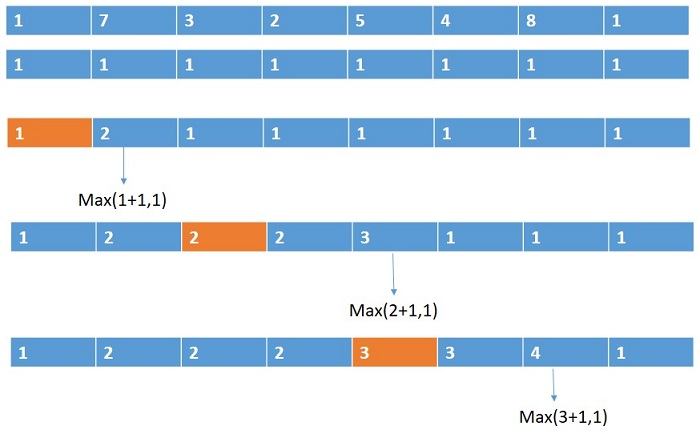2. Calculate the longest increasing subsequence from the rear of the array.
3. Add up the value of the two arrays and minus by one because in the first longest increasing sequence it is counted and next time from the rear it also counted.
4. Take the maximum value from there.
5. After getting the value we first find out the elements before it and then the last elements.
6. We go up for the elements those have a LIs value just less than of the current value and similar approach for the rear elements also.

For this sequence: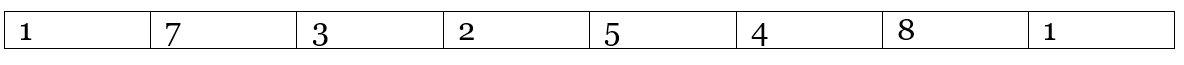Longest increasing subsequence from front: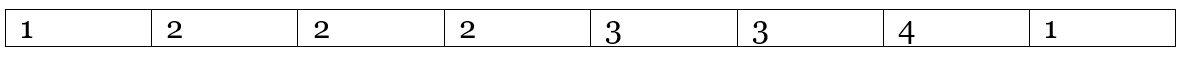Longest increasing subsequence from rear: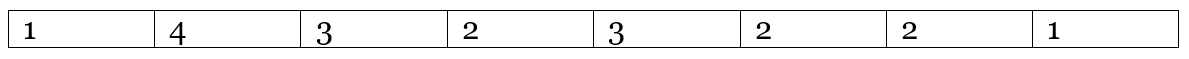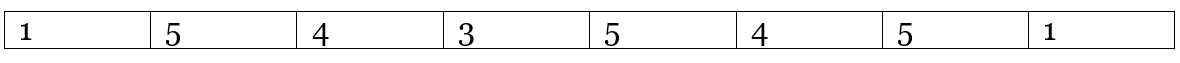Therefore, the length of the longest bitonic subsequence is 5.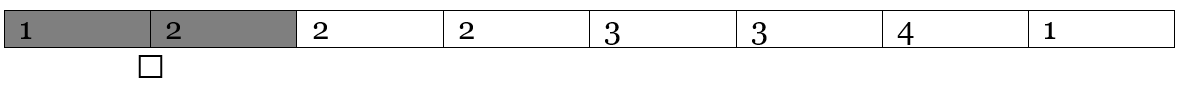Therefore, the numbers from the first LIS are: 1 7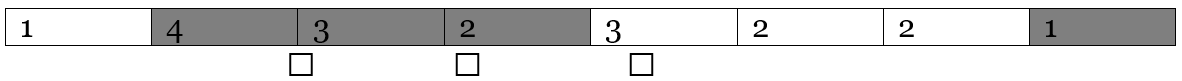Therefore, the numbers from the second LIS are: 3 2 1
Therefore, the sequence will be 1 7 3 2 1

C++ implementation:

```#include <bits/stdc++.h>
using namespace std;

void print_biotonic_sequences(int* f, int* l, int ind, int* a, int n)
{
int i = ind;
int j = ind;

list<int> lst;
lst.push_back(a[ind]);

while (i >= 0) {
//taking the ith element which have a diffencence of one from ind
if (a[i] < a[ind] && (f[i] + 1 == f[ind])) {
lst.push_front(a[i]);
ind = i;
}
i--;
}

ind = j;

while (j < n) {
if (a[ind] > a[j] && (l[j] + 1 == l[ind])) {
lst.push_back(a[j]);
ind = j;
}
j++;
}

list<int>::iterator it;

//print the values
cout << "The values are : ";
for (it = lst.begin(); it != lst.end(); it++) {
cout << *it << " ";
}
cout << endl;
}

int main()
{
int t;

cout << "Test Case : ";
cin >> t;

while (t--) {
int n;

cout << "Number of elements : ";
cin >> n;

int a[n];

cout << "Enter the elements : ";
for (int i = 0; i < n; i++) {
cin >> a[i];
}

int f[n], l[n];
//initiate the new arrays
for (int i = 0; i < n; i++) {
f[i] = 1;
l[i] = 1;
}

//calculate the LIS from front
for (int i = 1; i < n; i++) {
for (int j = 0; j < i; j++) {
if (a[j] < a[i])
f[i] = max(f[i], f[j] + 1);
}
}

//calculate the LIS from behind
for (int i = n - 2; i >= 0; i--) {
for (int j = n - 1; j > i; j--) {
if (a[j] < a[i])
l[i] = max(l[i], l[j] + 1);
}
}

int m = 0;
int store = 0;

//adding the two arrays to join them
for (int i = 0; i < n; i++) {
if (m < (f[i] + l[i] - 1)) {
store = i;
m = f[i] + l[i] - 1;
}
}
print_biotonic_sequences(f, l, store, a, n);
}
return 0;
}
```

Output

```Test Case : 3
Number of elements : 5
Enter the elements : 1 4 2 5 7
The sequence is : 1 2 5 7
Number of elements : 8
Enter the elements : 1 7 3 2 5 4 8 1
The sequence is : 1 7 3 2 1
Number of elements : 17
Enter the elements : 1 10 9 3 5 4 11 7 4 8 9 6 19 5 7 8 2
The sequence is : 1 3 4 7 8 9 6 5 2
```

What's New (MCQs)

Top Interview Coding Problems/Challenges!

Languages: » C » C++ » C++ STL » Java » Data Structure » C#.Net » Android » Kotlin » SQL
Web Technologies: » PHP » Python » JavaScript » CSS » Ajax » Node.js » Web programming/HTML
Solved programs: » C » C++ » DS » Java » C#
Aptitude que. & ans.: » C » C++ » Java » DBMS
Interview que. & ans.: » C » Embedded C » Java » SEO » HR
CS Subjects: » CS Basics » O.S. » Networks » DBMS » Embedded Systems » Cloud Computing
» Machine learning » CS Organizations » Linux » DOS
More: » Articles » Puzzles » News/Updates

© https://www.includehelp.com some rights reserved.